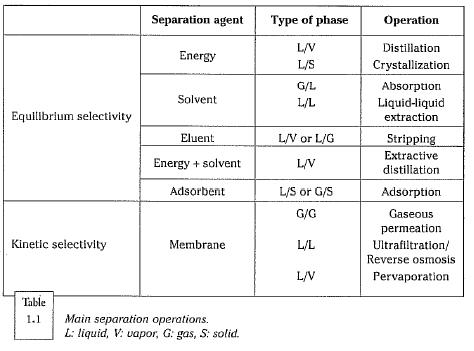﻿ Thermodynamic Equilibrium and Kinetic Factors – Oil & Gas Industry Technology Updates

## Thermodynamic Equilibrium and Kinetic Factors

Table 1.1 shows that the most common separation operations are based on equilibrium selectivity, also termed thermodynamic selectivity.This selectivity is due to the fact that at thermodynamic equilibrium, the distribution of the component(s) to be separated out is different in the exist­ing phases. The difference is related either to the thermodynamic properties of the relevant pure substances (boiling, or possibly crystallization, point) or to a difference in the components’ affinity with a selective separating agent such as a solvent or an adsorbent that has been added to the system. This is how a polar solvent can be used to separate aromatics from paraffins.

In a diagram with coordinates C, concentration in phase 1 and C, concen­tration in phase 2, the equilibrium equation is represented by a curve going through the origin (Fig. 1.6). The concentrations are expressed either in kg/m3 or in mol/m3, or expression can be considered in terms of mass fractions or mole fractions. Thermodynamic models generally use equations expressed in mole fractions. In contrast, when concentrations are used, it is easier to take kinetic factors (molecular diffusion) and hydrodynamic factors (such as axial mixing) into account. When the properties of the two phases are known, espe­cially the composition and the molar volumes of the components, it is possi­ble to go from mole fractions to concentrations and vice-versa.

For low concentrations, the equilibrium curve can be identified with its tan¬gent at the origin and thermodynamic equilibrium is characterized by a partition coefficient, defined as the ratio of the concentrations at equilibrium.